# The Foundations of Mathematics and Mathematica

Videoconference talk given at the International Mathematica Symposium (Hagenberg, Austria) August 23, 1999.

Well I’m very happy to be here today, if only in virtual form.

I guess when this conference was first being planned, I had hoped very much to be able to come also in physical form.

But as some of you probably know, for the last eight years I’ve been doing a very very big science project, and to get it finished, particularly recently, I’ve had to become more and more of a recluse.

In fact you would probably be surprised at how little I’m getting out now: in fact the very last conference I travelled any distance to was the IMS in Southampton four years ago.

So anyway I’m very much looking forward to having my science project finished, and being kind of free again.

And it’s perhaps because I’ve been a recluse so long I’ve decided that here I would try to talk about some somewhat advanced and abstract things—about the foundations of mathematics, and their relationship to my big science project, and their relationship to Mathematica.

Some of you probably know a little about what my science project is about—though I haven’t talked much about it. What I’m doing is something very ambitious.

I’m basically trying to build a whole new kind of science, certainly as big as any of the existing sciences, like physics and chemistry and things, and perhaps in some ways bigger.

The basic point of the science I’m trying to build is this. If you look at the history of science for the last 300 years, it’s probably fair to say the hard sciences have been following one basic idea: to find mathematical equations that represent things in nature.

Well, that idea worked pretty well for Newton and friends in studying orbits of planets and things like that. But in lots of other cases—for example in biology—it’s really never worked at all.

So the question is: what else can one do?

Well, I think it’s reasonable to assume that nature follows definite laws, definite rules: otherwise we couldn’t do science at all. But the question is: why should those rules be based on the constructs that we happen to have come up with in traditional mathematics?

Why should they be about numbers, and derivatives, and all those kinds of things in mathematics?

Well, what I decided to try to do nearly 20 years ago now was to see what science would be like if one thought about using more general kinds of rules: the kinds of rules that can easily be embodied in computer programs, but can’t necessarily be represented easily in traditional mathematics.

Some of you probably know some of the things I did in the early 1980s on cellular automata, and getting the field of complex systems research started. And I’m pretty happy with the work I did then, though I’m actually far from thrilled with the way the field developed in general terms afterwards.

I figured out quite a lot of stuff in the early 1980s: enough to convince me that the idea of generalizing the kinds of rules one uses to study nature isn’t completely crazy.

But then in the mid-1980s I got kind of stuck—I didn’t have very good tools to use for my computer experiments, and I seemed to be spending all my time writing little pieces of code and gluing them together and so on. And it was about then that I had the idea that perhaps I should build a big software system that would be able to do all the things I needed, and might even be useful to some other people as well.

And that was kind of one part of how Mathematica came to be. Of course since I’m a practical fellow, I tried to design Mathematica to be as useful as possible to as many other people as possible, too.

But a big part of my motivation for building Mathematica was that I wanted to use it myself.

And I’m happy to say that starting just after Version 2 came out, I was able to start doing that very seriously.

Well, I’ve discovered a huge huge amount of science with Mathematica. And I’m very much looking forward to telling everyone about it. But it’s a big intellectual structure that I’ve been building, and I’m not quite ready to talk about all of it yet.

I’ll talk about a few pieces here. And I hope that when my book about all of this—it’s called A New Kind of Science—is out you’ll all have a chance to really read about it more completely.

Well, what I want to do today is actually to talk about a topic that’s sort of at the intersection of my two most favorite kinds of topics: my new kind of science and Mathematica.

It turns out that at that intersection are questions about the foundations of mathematics, and there are some new things I’ve realized about the foundations of mathematics from working on my new science, and also from working on Mathematica.

I’m particularly happy to be talking about this here because there are several people in the Mathematica community who I’ve really enjoyed interacting with on these topics—though they definitely don’t always agree with me—particularly including Bruno Buchberger, Dana Scott, Klaus Sutner and Greg Chaitin.

OK, well what I’m going to say here may be somewhat abstract, but I hope and believe that with all of your background in Mathematica, you’ll be almost uniquely in a position to really get something out of what I’m trying to say. So let me try to get started.

I’ll tell you a little bit about my new kind of science, then I’ll tell you how it relates to the foundations of mathematics, and Mathematica.

There’s some pretty big shifts in intuition involved in my new science, and I certainly won’t be able to explain all of it here. But to understand the rest of what I’m going to say, I have to spend a few minutes explaining some of what my science is about.

Well, as I said, what my science is based on is thinking about what arbitrary sets of rules do: in effect, what arbitrary simple computer programs do.

Now normally when one builds computer programs, one sets up the computer programs to do specific things. But what I’m interested in in my new science is what arbitrary computer programs—say ones one might choose at random—do.

It’s very hard—in a sense provably impossible—just to figure this out by pure thought.

But it’s easy to do experiments. And what’s actually particularly fun about these experiments is that they’re so easy that they could even have been done probably thousands of years ago.

Maybe they were. I actually don’t think so. Because I think if people had ever seen the results they give then science would have actually developed along a very different track than the one it’s actually followed.

OK, so let’s take a look at these experiments, and let’s look at what some simple programs do.

I’m going to talk first of all about some things called cellular automata, because they happened to be the first things I looked at in the early 1980s. But one of the things I’ve discovered in the last eight years is that nothing I’m saying is really in the slightest bit specific to cellular automata.

OK, so what is a cellular automaton?

One can set one up by having a line of cells, each one let’s say black or white.

And then one has a rule for evolving the thing down the page. So let me show you an example.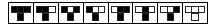So this might be a rule for the cellular automaton.  It says if you have a cell, with a black cell on the left or a black cell on the right, then that cell will become black on the next step.

So let’s ask what happens if we take this particular rule and just start it off from a single black cell.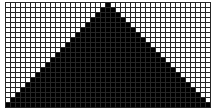OK, so this is what we get: it’s very straight forward. We start off with a single black cell at the top there, we apply this rule over and over again, and we just get a simple uniform pattern.

OK, well let’s try changing that rule a little bit and see what happens to the kind of pattern we get.  Here’s a rule, very similar to the previous one, yet slightly different.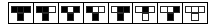Let’s see what that rule does.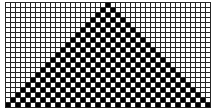So what we see is a checkerboard pattern.

Well, at this point we might guess that there’s some kind of theorem. And the theorem might say if you have a sufficiently simple rule, and you start it off with a sufficiently simple initial condition, then what you ‘ll get is a simple, let’s say periodic or repetitive, pattern.

Well, let’s try another rule. We can just change the rule we’ve been using a little bit.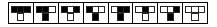Let’s try this rule here. Let’s see what pattern that produces.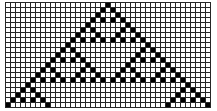Well that produces a rather different pattern.  What’s this pattern going to do?  Let’s run it, let’s say for 100 steps. Here’s the result that we get.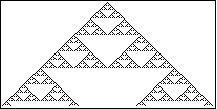And what we see here is that this particular cellular automata rule, when it starts off from a single black cell, instead of just making a repetitive pattern, it makes a nested self-similar pattern. This is one of those Sierpinski gasket fractals.

Well, just to emphasize how simple a kind of program a cellular automaton actually is, we could for example just write a cellular automaton program in Mathematica. Let me show you what a step of cellular automaton evolution looks like in a modern Mathematica. Here it is.

`Sign[BitAnd[2^ListConvolve[{1,2,4},a,2],num]]&`

So here for example, a is the current state, and num is the rule number. So here for instance, this was rule number 90. Let me regenerate the picture that I had above, by just running this little ListConvolve thing, which is the cellular automaton rule.

```Show[Graphics[Raster[1-Reverse[NestList[Sign[BitAnd
[2^ListConvolve[{1,2,4},#,2],90]]&,IntegerDigits
[2^25,2,51],25]]]],AspectRatio->Automatic,
Frame->True,FrameTicks->None];```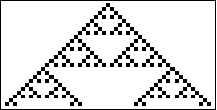Looking at what we get here, with our simple cellular automaton rule, starting off from a simple initial condition, we again get a pattern that we can recognize as quite simple. It’s a nested pattern.  So again we might guess that the true theorem is if you start off with a  simple rule and a simple initial condition, then you either get a repetitive pattern or a nested pattern.  But we might guess—and certainly that was my original guess—that if one has a sufficiently simple setup like that then there’s nothing more complicated that one can get.

OK, well let’s try this particular creature here. Here’s another cellular automaton rule, same kind of idea as the previous ones but different  particular choices of outcomes for different configurations.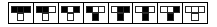Let’s see what this does.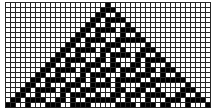Well this does something very strange. We’re starting off from just a single black square here, but now instead of  making something that looks like it’s obviously a repetitive pattern or a nested pattern, it looks like some more complicated mess.  Well, let’s go on. We can run that rule for a bit longer, see what it does.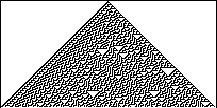It doesn’t seem to do anything terribly simple. We can keep running it. Let’s keep running it let’s say for 400 steps. Here’s the pattern that we get.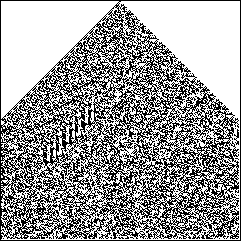Well you can see there’s some regularity in all this. But much of it is pretty random. And actually I know rather well that it’s random, because essentially we’ve been using the center column of this pattern as the thing that makes integer random numbers in Mathematica for the last 11 years. And so a lot of people have tested it, and nobody’s ever found any deviation from randomness.

But let’s just look at this picture.

It’s just nothing like we would expect.

I mean, from building things ourselves and doing engineering and so on, we’re used to the idea that if we want to get something complicated we’d better have a complicated set of plans or rules—or else we’ll just get something very simple out. But here we have some simple rules, there they are, and we start off from this very simple initial condition, yet we get something very complicated out.

So the question is: what’s going on here?

Well, I think what’s going on is that we’re basically seeing in a very direct form what I think is essentially what has been sort of the big secret of nature for a really long time.

You see, there’s a funny thing. If you look at two objects: one’s an artefact, one’s something made by nature, it’s a pretty good heuristic that the one that looks more complicated is the one that nature made. And the one that looks simpler is the one that humans made.

And I suppose it’s kind of that sort of thing that made people assume that there had to be some kind of superhuman supernatural intelligence behind the things that got built in nature.

But one of the things that’s happened in the past few hundred years is that it’s been pretty much figured out what a lot of the underlying rules in nature are. And actually they’re really pretty simple.

So that makes it even more bizarre that nature can produce all the complicated stuff we see.

And it’s been a big mystery how that can possibly happen.

But I think—and actually now I’ve accumulated a huge amount of evidence for it—that this thing that I’ve seen in simple programs like the cellular automaton that’s called rule 30, is the key to what’s going on.

See, the rules that we use for engineering are essentially special ones that we’ve set up to be able to do the tasks we want to do. And to make that work these rules have to produce only fairly simple behavior—behavior whose outcome we can reasonably foresee.

Otherwise we wouldn’t be able to use those rules to achieve particular tasks. But the point is that nature doesn’t have any constraint like that. So it can effectively pick its rules much more at random.

And the big fact that I’ve discovered is that if one does that a lot of the rules one sees—even if they themselves are very simple indeed—can produce incredibly complicated behavior.

And I think that’s basically what the sort of underlying secret of nature is.There are a huge number of questions in all sorts of areas of science that one can tackle for real once one knows this. And that’s part of what I’ve been doing for the past eight years.

But this phenomenon is also important for thinking about mathematics. In fact, I think the general approach I’ve been taking should lead not only to a new kind of science, but also to something that’s essentially a major generalization of mathematics. And that’s a large part of what I want to talk about here.

Well let me explain why that is.

One can think of mathematics as being something that effectively tries to work out the consequences of abstract sets of rules.

The question is what kinds of rules one uses.

And I think what’s happened is that mathematics has ended up using only rather special kinds of rules.

But in fact there’s a vast universe of other rules out there, which mathematics has essentially never looked at.

And actually the character of what those rules do, and what one can do with those rules, is somewhat different from what happens with the ones that are usually dealt with in mathematics.

I should say that if one wants to, one can certainly write a cellular automaton like the one I was showing there, in sort of mathematical form. This is in fact its rule. If P, Q, and R are the colors of neighboring sites, this is the rule for the color of the next site.

`Mod[p+q+r+q r,2]`

But it turns out that even though one might be able to state this as a mathematical rule, it’s not a terribly natural thing to do.  And the usual ideas of for example algebra that one might try to apply to something like this, tell one almost nothing about the behavior that one gets from the rule.

So effectively what’s going on here is something that ends up being quite disconnected from the kinds of things that we usually think of as mathematics.

OK, so one question is, if mathematics isn’t dealing with genuinely arbitrary abstract rules, what exactly is it dealing with?

I think in the end to figure this out, one effectively has to look at history.

I think defining mathematics is a little like defining life. One knows definitions of life that say something is living if it can move itself around, or if it can reproduce itself, or if it eats things, and so on. But as one goes on and looks at all these definitions, one realizes that there are lots of devices, physical processes and so on, that satisfy all of these various definitions, just like life does. And in the end the only definitions that actually work and correspond to what we intuitively think of as being a living system, are ones that involve the specifics of containing DNA and things like that. And essentially involve the particular history that life has taken on Earth over the course of geological time.

And I think it’s sort of the same with mathematics. One can come up with all sorts of abstractions for what mathematics might be, but in the end one basically has to look at history.

And essentially one has to go back to things that were happening in ancient Babylon.

What seems to have happened in Babylon is that two things were developed: essentially arithmetic, and geometry.

And I think that what’s happened is that since then essentially all the mathematics that’s been developed has somehow been derived from those original forms of arithmetic and geometry.

Actually if one looks up the definition of mathematics in most dictionaries, one will find a definition that basically agrees with this.

But it’s not the definition that most professional pure mathematicians imagine is the appropriate one for mathematics. They think that somehow mathematics is about studying quite arbitrary abstract systems.

And that’s actually  how I thought about mathematics when I named Mathematica  "Mathematica".

But if you actually look as a practical matter at what mathematics is as mathematicians actually do it—how that’s defined—it’s somewhat different.

See for a long time in the history of the development of mathematics, people thought of mathematics as somehow being about describing the way our universe works. And that was the justification that was used for the kinds of constructs that were developed in mathematics, the ones that should be considered, and the ones that should not be considered.

And that’s what led for example historically to the fact that the idea of having more than three dimensions to space was a hard thing to introduce, and things like that.

And—particularly after Newton and friends did their stuff—it was kind of felt that for sure the generalizations of arithmetic and geometry that have been so successful in their efforts to kind of put a formalism into science should be enough to describe everything that’s relevant in our universe.

But one thing I can tell you pretty definitively from the science that I’ve done is that that’s just not true.

And in fact it’s that assumption that’s made a lot of science get stuck.

A big part of what I’ve discovered is that the kinds of rules that nature really seems to follow are ones that are pretty easy to represent in simple computer programs, but almost impossible to represent in traditional kind of arithmetic-and-geometry mathematics.

So the idea that one shouldn’t make the rules in mathematics more general because that would mean going beyond anything that’s in our actual universe is just wrong. That’s not a reason to not think about generalizing the kind of arithmetic and geometry-based rules in mathematics.

Of course, people in mathematics kind of thought they stopped worrying about making rules correspond to our universe about a century ago.

That was one of the big achievements of Cantor, and to some extent Galois, and friends. To start making constructs in mathematics that were purely abstract, and not intended to be linked to anything in the actual universe.

But OK, without the constraint of linking things to what happens in the actual universe, how do people pick the constructs to use in mathematics?

Well that’s an interesting story and it’s sort of central to what mathematics is, and what it isn’t.

And it turns out that what it all revolves around is the idea that mathematicians, at least in the last century or so, seem to have been proudest of: the idea of proof in mathematics.

I guess that mathematicians always feel that they want certainty; they want to be sure that they are figuring out things that are true.

And they have the idea that the way that one does this always has to do with proofs.

I guess that idea started with Euclid and friends. Euclid didn’t want to rely on how some geometrical figure was drawn in the sand or whatever, and whether two angles that looked like they were the same, were the same as far as he could measure them. He wanted to know precisely were these two angles the same, not just did they look the same as well as he could measure them.

He wanted a proof, some kind of logical proof, that these two angles were exactly the same.

So he got into the idea of essentially using logic to take some statement and see if it could logically be deduced from some set axioms.

Now, to be fair, not all mathematics has historically done that way. Particularly in areas like number theory, people—people like Gauss for example—quite often did experiments to see what was true.

But particularly as things got more hairy with infinitesimals, and things where everyday intuition seemed never to work, it got pretty much taken for granted that proof was the only way to move forward in mathematics.

And in fact if you look at the Bourbaki books, for example, their opening words are: "Since the time of the Greeks, to say ‘mathematics’ has meant the same as to say ‘proof’".

Well, I myself have never been a great fan of the idea of proof.

And perhaps that shows that I really am a scientist, not a mathematician. I like starting out without knowing what’s true, and making observations and doing experiments, and then finding out what’s true. Sort of discovering things from scratch.

I don’t like somehow having to guess what’s true, then work backwards and try to find a proof of it.

And actually my experiences have kind of strongly conditioned me this way by now. Because in the science I’ve done I’ve discovered—by being I think a fairly careful experimenter—things that I’d never possibly have guessed.

And actually I’ve discovered quite a few things that I at least had thought I had proved were impossible.

Of course, once I actually found out what’s true, I could see that there were bugs in the proof.

But at least until we have things like Bruno’s Theorema up and running there’s no way at all to detect those bugs. It’s not like Mathematica, where we can do all kinds of automated software quality assurance and so on.

We have to rely on human reviewers in journals and things like that, which is a very unreliable basis I think for finding out what might be true.

Well even though I myself don’t happen to be particularly thrilled with it, mathematics as an activity has taken proof pretty seriously.

In fact, it’s taken it so seriously that it hasn’t really looked much at areas where it seems like you can’t do proofs easily.

And essentially I think that’s why there’s never been mathematics that’s looked for example at my friend the rule 30 cellular automaton.

In fact, I think it’s a pretty good model for how mathematics has grown—that there’s a pretty good model that one can make that’s pretty much this.

It all started sometime in ancient Babylon from plain arithmetic and geometry. And those in turn actually arose from practical applications in commerce and land surveying and so on.

And in these areas, some neat theorems were found, and some proofs were developed for those theorems.

And being extremely pleased with the theorems, mathematicians started looking for ways to get the most out of these theorems.

And to do this, what they tended to do was to generalize the constructs that these theorems dealt with in such a way that the theorems always stayed true.

So the idea was to make things as general as possible, making that generalization by having the constraint that some theorem or another could still be proved, but then sort of take away as many pieces as possible while still letting the theorem be proved.

So of course with this scheme one thing one can be sure of is that the systems that one was going to set up, were ones where the idea of proof still worked.

Well, one of the questions that’s often asked is a question about whether mathematics is sort of invented or discovered.

I think it’s pretty clear that the sets of rules that mathematics has actually ended up looking at were very much invented—in a definite human way, with particular constraints.

I suppose it’s kind of weird: one usually thinks that mathematics is somehow more general and more abstract than, say, physics, or some other kind of natural science.

And the reason for that is that one thinks its rules are somehow more arbitrary.

It’s somehow dealing with more arbitrary kinds of rules.

But actually the conclusion that I’ve come to is that that’s not true, and that in fact the rules in physics—while there are many fewer of them—are chosen in a sense more arbitrarily—and are probably much more representative of all possible rules than the ones that have typically ended up being studied in mathematics.

So, in a sense, my adventures in looking at what arbitrary programs do can be thought of as a big generalization of ordinary mathematics—in a sense a study of what arbitrary mathematicses in the space of all possible mathematicses do.

Well as I’ll talk about a little later, an arbitrary mathematics has some similarities to our particular human mathematics—the one that’s developed historically—but it also has  some pretty substantial differences.

But before I go on and talk about that, let me say a little about how all this relates to Mathematica, and to thinking about what one can call the foundations of Mathematica.

Well, first let’s sort of say what my kind of abstract view of Mathematica is.

I’ve kind of viewed my job in designing Mathematica to be to think about all possible computations that one might want to do, and then to identify definite chunks of computational work that would get done over and over again in those computations.

And then what I’ve tried to do is give names to those chunks. And those are the primitive functions of Mathematica.

Well, one of the things I’ve learned from building my new kind of science is that I did a decent job in a certain way: the constructs that I put into Mathematica correspond pretty well with the constructs that seem to be relevant for representing for example the rules in our universe and in the natural world.

That has a very practical consequence.If you look at my new book when it’s out, you’ll find the notes in the back are full of Mathematica programs. And those programs implement the various kinds of programs that I talk about in the book.

But the point is that those implementations are mostly very very short. In other words, it doesn’t take many Mathematica primitives to build the kinds of constructs one needs to represents the things that I think are going on in our universe.

Here’s an extreme example of that: one of the things I’ve been doing a bit of is taking what I’ve discovered, and trying to use it to finally come up with a truly fundamental theory of physics. In a sense I’ve been trying to reduce physics to mathematics: to get a single abstract structure that is, exactly, everything in our universe.

That sounds like an extremely tall order, but for reasons I’m not going to go into here, I’m increasingly optimistic about my chances of success.

But the point is that one of the ways one can measure how well the primitives in Mathematica were chosen is how complicated the final program that is the universe needs to be, when it’s written in Mathematica.

And actually, I had thought it was going to need to be rather long. But I noticed a year or so ago that in fact the basic system I was studying could actually be written as a surprisingly short collection of somewhat bizarre rules for Mathematica patterns.The only problem was that they didn’t run all that fast.

I suppose that’s not surprising if you’re trying to reproduce the whole universe, that it not run all that fast.

But in the next version of Mathematica, or perhaps the one after that, I will tell you now the slight secret that there’ll be a few hidden optimizations that make the universe run a little faster in Mathematica!

Well, I’m pretty confident that the primitives in Mathematica are well chosen if one wants to represent fairly arbitrary computations of the kind that for example seem to get used in natural science.

But what if one wants to "do mathematics"? Are the primitives that one has the best possible primitives?

Well, obviously one can go a very long way with these primitives, as we’ve all done.

And actually that right there tells one something about what’s involved in mathematics.

But obviously there are a lot of primitives in Mathematica—say things like Factor and Expand and so on—that are very specifically set up to fit into particular kinds of structures that people often use in mathematics.

And then way down at the bottom level in Mathematica there are general transformation rules, which seem to be a good representation for arbitrary computations.

But the question is: what are the intermediate constructs that are not specific to particular areas of mathematics, but are constructs that support the general kinds of rules that are used in mathematics?

Well, to answer this one has to know more about what mathematics actually  is, and what special characteristics its rules have.

One might think that the question of "what is mathematics?" is just one of these abstract things only of interest to philosophers.

But actually if one’s trying to design the most general stuff that will be useful in Mathematica, for mathematics, it becomes very important in practice.

And I should say immediately that I haven’t figured the answers here out, though I think I’m now beginning to define at least some of the relevant questions.

I kind of like these kinds of problems; in fact, in a sense this is ultimately the kind of thing I’ve spent most of my adult life doing: Trying to kind of whittle things down to find the very simplest most minimal constructs that one needs to do things.  Whether that’s cellular automata and models of nature, or whether that’s constructs to support programming in Mathematica.

Of course it’s the fate of someone like me who spends a huge amount of time figuring out things like this that all these things in the end come to seem obvious.

Actually I’ve often thought how terrific it would be if people would study a little more carefully why things in Mathematica for example are done the way they are. There’s often a huge amount of thought behind things that might seem obvious, and having that thought more widely understood would be really good for everyone.

Anyway, I’m getting off-topic.

I was talking about understanding what the essential features of the activity that we call "mathematics" really are.

So how does one start on a question like this? Well, like any natural scientist I think the place to start is to build a model.

By the way, just in case there are any official mathematical logic model theorists in this audience, I don’t mean quite your kind of model. I mean the kind of model a physicist for example builds.

And the essence of that kind of model is that it’s somehow an idealization of a system.

What one tries to do in making a model in natural science is to capture those aspects of a system that one cares about, and ignore all the others. A model is in the end an abstract thing. I mean, one doesn’t think a planet going around its orbit has a bunch of little cogs—or little Mathematicas—inside it solving differential equations.

Instead, the differential equation is an abstract representation of the effect of what happens.

I mean, the number of times I’ve heard physicists say: but how can your cellular automata model be a model for such-and-such, because we know the thing itself is solving a differential equation?

Well of course that’s not true, both the differential equation and the cellular automaton are just abstract representations of an idealization of what the system is doing.

The bottom line is that a model, as that term is used in natural science, is an abstract idealization of something.

OK, so what’s a good model for mathematics?

Well what we need is something that captures the essential features for our purposes of mathematics, but leaves all the inessential details  out.

Well, I think it’s been fairly clear for a century or so that the first step is to think about mathematics in terms of operations on some kind of symbolic structures.

And there are areas of mathematics like category theory that go a certain distance in defining general operations on symbolic structures.

But I’ve never thought they go nearly far enough.

And when I was designing Mathematica what I ended up trying to do was to set things up so one could go much further . . . so one could set up absolutely arbitrary symbolic structures and do essentially completely general transformations on them.

I originally came to this—actually when I was working on SMP around 1979—by thinking about how to capture and generalize what humans do when they’re doing mathematics by hand.

I sort of imagined that one was always looking up books and finding rules that said that an expression like this gets transformed into an expression like that.

And then I kept on generalizing that idea, and ended up with the notion of transformation rules for patterns.

And as we all know from Mathematica that idea is extremely successful in representing all kinds of computational and mathematical ideas. And gradually more and more people even seem to understand that point.

Well, what I did with transformation rules in SMP and later Mathematica is a bit like the whole tradition of work in mathematical logic and in the theory of computation.

But it’s different—at least in intent—in some subtle but rather important ways.

And to understand more about what people usually think of as being the discipline of mathematics I’ll have to explain something about that.

Ultimately the big difference is between being interested in doing proofs and being interested in doing calculations.

But to see what’s gone on I’ll have to tell you again a little bit about the history of all this.

Well, I guess it was at the end of the 1800s, particularly following all the discoveries made by generalizing Euclid’s axioms and so on, there got to be the idea that somehow mathematics could be thought of as a study of the consequences of appropriately chosen sets of axioms.

And it turns out that axioms in this sense are very much like transformation rules for symbolic expressions.

These are Peano’s axioms.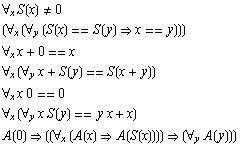So this is just an example of an axiom system.  These are Peano’s axioms for arithmetic. And one can see that they’re—I’m not going to go into this in great detail—one can think about these things as essentially like transformation rules for symbolic expressions.

Well actually, in the way things are often set up, there are usually so-called "rules of inference" in addition to axioms. And it’s these rules of inference that are the direct analog of transformation rules.

But actually, as I’ll perhaps explain later, one can just as well think about the axioms themselves as being like transformation rules.

And essentially what the axioms say is that one mathematical construct or statement can be transformed into another mathematical construct or statement.

OK. Well that sounds very much like transformation rules in Mathematica. But there’s a crucial difference, and it’s associated with the word "can".

You see, in Mathematica, one just takes an expression and then uses a sequence of transformation rules and looks at what comes out.

But in the axiom setup in mathematics, one is usually interested in asking whether one can find any sequence of transformation rules that will get one from one particular expression to another.

It’s basically the difference between calculation and proof.

In a calculation one just wants to follow a procedure and get an answer.

In a proof one wants to know whether there’s any path one can follow that goes from one statement to another.

And as a practical matter it’s usually much easier to do calculations than to do proofs.

And that’s sort of why Mathematica can work well, and why logic programming languages like Prolog and so on that are closer to emulating proofs never really work that well.

And that’s why I’ve always tried to be very careful in designing Mathematica to set modest expectations with the names of functions like Simplify that have to do things that are more like proofs.

But anyway, the idea of thinking of mathematics as the study of the consequences of axiom systems did introduce the notion of transformation rules historically.

But so what were these transformation rules like?

Well, actually, some of them were actually formulated very much like the ones in Mathematica.

And in fact by the 1940s there were transformation rules being talked about that are very similar in spirit to the ones in Mathematica.

But at least when people thought about these being applied to mathematics, they normally thought about them as being used in a proof kind of way rather than in the kind of way they are used in Mathematica.

OK, so basically what we’ve learned here is that the kinds of transformation rules that are in Mathematica are decent models for the kinds of transformation rules that are in mathematics.

And of course in a sense the success of Mathematica already told us that.

But one question is, do we need all the complicated stuff that’s in the transformation rules in Mathematica to be able to reproduce what are somehow the essential features of mathematics?

Well, I don’t think so. Not at all in fact.

And actually that’s analogous to what I’ve found over and over again in physics and biology and other natural sciences.The really important and general phenomena don’t depend on very much, so one can perfectly well capture them even with very simple models.

OK. So what then is the appropriate minimal model for mathematics?

What can we get rid of and still have the most important phenomena in mathematics?

Well, Mathematica patterns have already gotten rid of all sorts of hair that one usually sees in formulations of mathematics.

For example, there are no explicit types in Mathematica expressions. Only implicit ones from the structure of the expression tree or the names of heads.

I might say that when Mathematica was young, people often said: you can’t do anything like mathematics without having an explicit notion of types.

But actually I think it’s fairly clear that they were wrong. What we can do in Mathematica with symbolic expressions and their structures is actually much more general than what one can do with ordinary types.

Let me make a brief historical digression, that some of you may find fun, about types.

Well, in an effort to build up all of mathematics from some kind of uniform set of primitives the first really serious efforts along these lines got made by Frege in the late 1800s and by Russell and Whitehead in the early 1900s.

All of these folks had the idea that one should start from logic to try to build up all of mathematics.

For various reasons, I actually happen to think that the basic idea is fairly silly—that one wants to start actually from much more arbitrary symbolic structures, rather than from the particular structure defined by logic.

But anyway, what they were trying to do was a little like what I’m talking about today in making minimal models for mathematics—or, for that matter, what I was trying to do in the underlying design of Mathematica.

They wanted to be able to have a small set of primitives, and then assemble these to represent all the constructs of mathematics.

Well, they had a difficult time doing what they wanted to do. And of course they had many practical disadvantages compared to what we can do today. Like never being able to run the things they created.

But all in all I’ve actually always considered Principia Mathematica of Russell and Whitehead to be perhaps the worst example of language design of all time.I think some modern languages and systems are pretty bad. But actually nothing compared to Principia Mathematica.

One of the most baroque pieces of Principia Mathematica in fact has to do with types—and later ramified types—which were originally introduced as a way to avoid various logical paradoxes and so on.

Well, anyway—it’s all a long story, not all of which I even know—but this idea of types that kind of came from this detail about avoiding paradoxes and this rather baroque formalism of Principia Mathematica somehow got assumed to be fundamental to all of the formalism of mathematics.

But I think Mathematica proves that it isn’t really  necessary to think about that kind of thing.

So, alright, let’s assume Mathematica transformation rules are enough to represent mathematics. But what can we drop then in these transformation rules?

Well, another big feature of transformation rules is variables, and the ways variables are treated in scoping and things like that.

Another big feature of transformation rules, at least in Mathematica, is that they operate on expressions that can be thought of a bit like trees.

But let’s for the time being assume we can just ignore both of these ideas.

Later, if there’s time, I can talk a little bit about some rather abstract things called combinators that actually don’t ignore these ideas, but still give very simple models of mathematics. But the models are a bit harder to understand than the models that I was planning to talk about.

OK, so we’re going to ignore variables and tree structures.

What’s then left in Mathematica and its transformation rules and so on?

Basically what Mathematica is then doing is transforming sequences of symbols, or strings of symbols.

Well, in a practical use of Mathematica the appropriate strings tend to be pretty complicated.

In an effort to find the minimal stuff that’s going on, let’s see what happens if we work only with very simple strings.

OK. So let me try and show you some examples of what an absolutely minimal Mathematica would look like.

Well, let’s formulate this in Mathematica. Let’s have an object s that I’m going to say is Flat.

I’m going to define various rules for s of various sequences of elements. And then all I’m going to do at every step is to apply these rules to the sequence of elements I’ve got, just following Mathematica‘s normal how-it-applies-rules scheme.

So let’s take the rule s[1,0]->s[0,1,0]. This says "1,0 gets turned into 0,1,0".

So let’s then apply the rule six times.

`NestList[# /. {s[1, 0] -> s[0, 1, 0]}&,s[1,0,1,0],6]`

OK? Let’s see what that does.

```{s[1,0,1,0], s[0,1,0,1,0], s[0,0,1,0,1,0],
s[0,0,0,1,0,1,0], s[0,0,0,0,1,0,1,0],
s[0,0,0,0,0,1,0,1,0], s[0,0,0,0,0,0,1,0,1,0]}```

Well, we can make a picture that corresponds to the outcome that I got here, and it says that at every step that rule just prepends a "0" onto the string that we have. OK?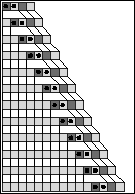Well so that’s an extremely trivial example of what Mathematica can do so to speak. Let’s try looking at another rule. Let’s look at this rule here. This rule has two pieces in it. But it’s, again, it’s the same structure of rule.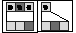Let’s see what that does if we just start it off from some string.  So here’s what that does after a few steps.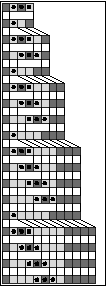So you can see it’s doing something a little bit more complicated. And again what this is doing is it’s just doing a sort of minimal version of what Mathematica does when it applies transformation rules. Here’s a slightly bigger version of what happens with that rule.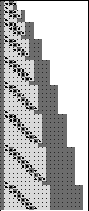So again, just like our intuition might have suggested, if we have sufficiently simple rules here, we’re doing the minimal version of what Mathematica does, the results are simple just like the rules are simple.

Let’s try another example. Here’s another rule.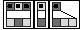OK? It’s slightly more complicated. Now there are 3 replacements in our list of Mathematica rules.  Let’s see what that one does.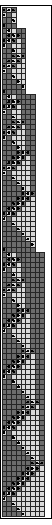A little bit more complicated.  You can just see it going down the left. It’s a little bit more complicated. You can see it’s doing this sort of nested, kind of fractally thing. But again, it’s comparatively simple.

OK, let’s try another example. This is another set of sort of minimal Mathematica rules. Pretty simple set of rules.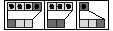We could just write it down in Mathematica notation so to speak. In  Mathematica notation, that rule would just be this thing here:

`{s[0,0,0,1]->s[1,0,0], s[0,0,0]->s[1,1], s->s[0,0,1]}`

Let’s see what that rule does if we apply it to some particular initial condition. Say we start off with "1 0 1".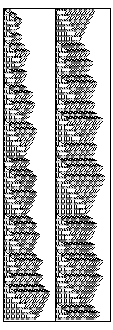OK, that’s what this rule does. Pretty simple rule as I showed, pretty simple initial condition. But that’s the result it generates. Let’s try running it for a bit longer.  So what I’ve done here by the way is I’ve kind of folded this around so it goes down the first column then up to the top of the second column, and so on. Let’s try running it, say just for a few hundred steps.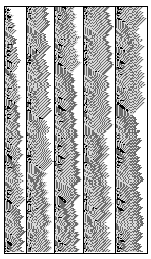There’s what we get.  Again I’ve folded these columns around. So this is again an example, just like in my rule 30 cellular automaton, of a case where we have very simple rules, we start off with a very simple initial condition, yet the behavior we get is something very complicated.

And notice that this is a minimal idealization of Mathematica. This is not something like a cellular automaton that we got from somewhere else. This is something that just comes from essentially looking at an idealization of Mathematica, and in a sense an idealization of mathematics.

So what can we say about pictures like this.

Well, one pretty much immediate thing that one can say is that it can end up being undecidable what actually ends up happening in a picture like this.

I don’t think I have time to explain lots about universal computation, and about a much stronger phenomenon that I call computational irreducibility.

But let’s see what it might mean to say that something is undecidable in a system like this one.

Let me use a cellular automaton as an example. Let me pick a particular cellular automaton and let’s start off running the cellular automaton with an initial condition that has just a single gray square in it. Well it’s very easy to decide what will happen to the cellular automaton. After a little while the activity will halt, and the pattern will die out.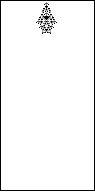But let’s say we change the initial condition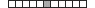a little bit. Instead of having just a single 1 [light-gray], a  2,3 [dark-gray cell, black cell] in the initial conditions.So that’s what it looks like then. It’s a lot less clear what’s going to happen then. Let’s run it for 300 steps for example.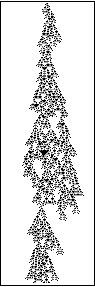Is it going to die out? Is it not going to die out?  How can we tell?  Let’s try running it for say 1200 steps. OK, and here’s the result we get.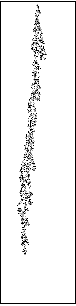Here’s the result we get from that . . . scroll down . . . . Well we see that sometime before 1200 steps, after doing all kinds of crazy things, this pattern eventually died out.

Well let’s take another initial condition, let’s say a pair of ones [pair of light-gray cells].Let’s see what happens under those circumstances. Here’s what we get then.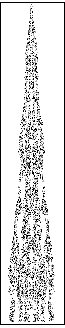And actually, I don’t know what happens in this case. I can follow it for perhaps a million steps or so and it’s still just kind of globulating around, and it sort of hasn’t made up its mind about what it’s going to do. It’s not clear whether it’s going to halt or not. And this is sort of a quintessential example of something where, the question of whether it ultimately halts or not, is something that will be formally undecidable. Well, how can one think about this?

If one thinks about trying to work out what will happen in a system like this, what one does when one tries to predict the system is one tries to have some kind of device that will outrun the actual evolution of the system. One can try to find some clever system that will be able to predict whatever the cellular automaton will do before the cellular automaton knows it.

But the best kind of predicting device will end up being a universal computer. It turns out that this cellular automaton itself is almost certainly a universal computer. For those of you who know about these things, that’s actually a rather surprising and stunning fact. But I won’t go into that right now.

Anyway that means that one will never be able to have a predicting device that will systematically outrun the actual cellular automaton.

So one will never be able to tell for sure what will happen after an infinite time, except in effect by spending an infinite time figuring it out, just like the cellular automaton does.

And that’s why one says that the halting problem of telling whether a pattern will ever die out is undecidable.

Well OK. So this undecidability thing also happens in the string rewriting systems that I talked about before.

And it also happens in dealing with transformation rules in mathematics. But the point is that one doesn’t need all the hair associated with actual ordinary mathematics to get this phenomenon. It already happens in our very simple idealization.

But back to the idealization.

This string rewriting thing that I’ve talked about is a decent idealization of Mathematica and of the calculational style of mathematics.

But what about the proof style of mathematics?

Well, it turns out to be pretty easy to get an idealization of that too.

The whole thing is that in the string rewriting system that I did above, I always did things in sort of Mathematica style.

At each step, I just scanned the string, and applied the first rule that I could.

So at every step, there was a definite answer.

But in doing proof-style mathematics, one wants to do something different.

One wants to make what I call a multiway system, where one looks not just at a specific outcome from doing string rewriting in a particular way, but instead at all possible outcomes.

So let’s see how that works. Here’s an example of a multiway system. So the idea is that it’s also a string rewriting system. This particular one uses these rules here—one square can get rewritten to one square, or one square can get rewritten to a pair of squares.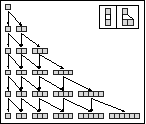But instead of just rewriting the string , instead of just keeping the first rewriting that we end up doing, what we do is to look at all possible rewritings of the strings. So that means every little box here corresponds to one of the strings that can be generated, and there are arrows joining the boxes to show what can be derived from what by doing rewritings.

OK, so let me show you another example just to make clear what I’m doing here.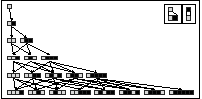This one that I have here is a kind of Fibonacci-style string rewriting system, where white gets rewritten to white-black, and black gets rewritten to white. And so it’s fairly easy to work out that there will be Golden Ratio to the n approximately—Fibonacci of n—strings, at each of the 1, 1, 2, 3, 5, 8 etc. distinct strings at each step.

So what’s the analog of a proof in this kind of system?

Well, it’s actually pretty simple. A proof is like a path on this network. A proof is something that shows one that one particular string can be derived from another string. Remember that the axioms were these transformation rules here, which showed how strings can be rewritten. And the point is that what one is trying to do when one derives theorems is essentially make up sort of super-transformation rules—things that for example say well if you have the string white-black-white, it can get rewritten to white-white-black-black-black down here.

Being able to do multiple steps, of this rewriting where the rewriting is done by the axioms. And one could then add that as an axiom, to say that white-black-white can turn into this thing and then see what could be produced that way, and in the end the set of strings that one would generate would be the same as the set of strings that one generates just by following these axioms, but one would be able to do it quicker.

So essentially adding theorems, deriving theorems and adding theorems allows one to collapse the network, and get new theorems more easily.

Well, what can one tell from looking at these multiway systems? One question is, what do they typically do? These ones that I’ve shown you here do pretty simple things. But let’s see what the typical multiway system does. One can sort of pick here essentially the axioms for the multiway  systems at random and one can ask, what then is the resulting behavior of the network of theorems associated with this multiway system. So here’s an example of a rule for a multiway system, and let’s see what that one does.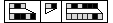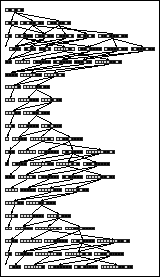That one actually does something quite complicated. It’s a pretty simple rule, yet again, like all of my things here. But what it does is quite complicated.

And, for example, I can ask many kinds of things about this system. For example I could ask, starting off from this particular initial string, "When does the string that just consists of a single square first occur?" And the answer is it takes quite a few steps before that short string first occurs. Actually if you look at all possible multiway systems, most either don’t generate many strings at all, or generate very rapidly increasing numbers of strings.

But it’s not hard to find these weird and funky multiway systems that don’t generate terribly many strings, but the number of strings they generate varies wildly with time. They don’t have to have terribly complicated rules, and so it’s fairly clear, from the fact that you can get multiway systems like this, that it ends up being essentially impossible to tell how long a chain of strings one will have to go through to get even from some fairly short string like this one some other short string like this one.

And that’s essentially directly analogous to the observation in mathematics that even pretty simple theorems can have very long proofs. But we’re already seeing that phenomenon in our extremely simplified model of mathematics.

And because we’ve got a nice simple model of mathematics, we can ask questions about what the distribution of lengths of proofs for propositions of certain lengths is.  For example, this is a picture that shows those strings which are eventually generated which correspond essentially to those propositions that turn out to be true, and that turn out to be provable. And it shows how far you have to go before you can generate those things.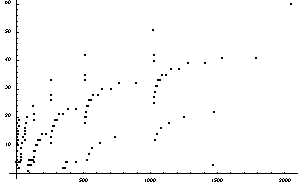I might say by the way, let me just show you another example of a slightly long proof in one of these systems. This is a written out proof in one of these systems.  This says you rewrite a white square to black-white-black, you then rewrite the white square in the middle there to black-white-black, in this way, and you’re kind of writing out a proof here, to show how you get from a white square to a black square.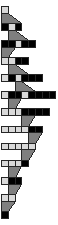And in this particular system there’s actually more than one way to get from a white square to a black square, actually these are all the possible shortest proofs that correspond to getting from a white square to a black square.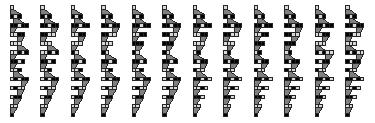OK. Let’s go back to talking about phenomena in mathematics and how they relate to phenomena in this kind of simplified  idealization of mathematics.

Well one very famous phenomenon in mathematics is Gödel’s Theorem. And I thought I might tell you a little bit about how Gödel’s Theorem is related  to what I’ve been saying.

Well I have to admit something about the models I’ve been using so far. They are a bit different from what people consider usually as mathematics, because they don’t have logic, they don’t have a notion of logic explicitly built into them. As I mentioned before, most of traditional and proof-based mathematics is ultimately based on logic.

I’m not sure that’s the best thing, but that’s the way it is.

The kinds of multiway systems that I’ve been showing you so far, are fine ways of representing relations in mathematics—but not quite theorems.

Actually some people may recognize my multiway systems as being things that are sometimes called semi-Thue systems.

And these are essentially semi-semi-groups.You see, you can think of the transformations that I’m doing here as being like relations between words in an algebraic structure. I’m going to get slightly technical here but it might be of interest to some people and help people to understand what’s going on.

In my systems the transformations can be absolutely anything. But if every transformation can be applied in either direction then it corresponds to the relations of a semigroup. And if in addition there are inverse elements allowed, one has a group. Now, being even more technical, I should say that the pictures I’m making you might think were Cayley diagrams, they’re not. They’re actually a kind of lower level construct. They’re pictures of sort of what’s inside each node of a Cayley diagram—of all the words that are equivalent, say, to the identity.

OK. Well, some of you may know about the undecidability of the word problem for groups.That’s essentially the same statement I’m making—about the paths that go from one word to another by applying transformations being arbitrarily long.

Let me talk about a more familiar example. Let’s think of the poor Simplify function in Mathematica. It works by applying various transformation rules to an expression. Let’s say that we’re trying to determine whether some particular expression is zero. Well, some transformations may make the expression smaller, but some may make it bigger. And we can end up having to go on an arbitrarily long path in the attempt to find out whether our expression is zero or not. And actually that’s the same phenomenon I’ve shown you too.

OK. But back to the questions about theorems. I’ve said that these multiway systems of mine don’t really have logic built into them.The underlying problem is that they don’t have explicit notions of True and False. They’re just dealing with expressions, and transformations between expressions. If those expressions are supposed to be propositions—candidate theorems in some kind of mathematics—they should presumably be either True or False.

So how do we set this up? Well, it’s really very easy. We just need some operation on strings that’s like negation, and that turns a True string into a False one and vice versa. And so as an example the operation might just reverse the color of each element.

OK. So now how do we find all the True theorems? Well, we just start from the string that represents True, let’s say a single black cell, and then we find all the strings that we can derive from it. But . . . there are some nasty things that can go wrong. If our rules—our axioms—aren’t sensible, we might be able to derive two strings that are related by negation. And that would mean that we have some kind of inconsistency. There are two theorems we’re saying are both true, but they are negations of each other.

And most mathematicians would then say that the axiom system one’s picked is no good. But let’s say we avoid this problem, and that we are sure nothing inconsistent like that happens.Then are all axiom systems with this property decent axiom systems for mathematics?

Well, there’s another problem. And that problem is completeness. Given a theorem, can we actually determine from the axioms whether the theorem is True or False? Well, in terms of our networks that’s an easy question to formulate.

We want to know whether anywhere on the network our particular proposition, our particular theorem will appear, starting from the string that corresponds to True. Well, it’s pretty obvious there are lots of axiom systems where tons of strings will never appear—axiom systems that are utterly incomplete. But what would a mathematician think of one of those axiom systems? They’d probably think for many purposes that they were kind of stupid.

Let’s say one had an axiom system that was supposed to have something to do with arithmetic. And it was all very nice. But it couldn’t prove that addition is commutative. Mathematicians would probably say that that was a dumb axiom system; that they need a more powerful axiom system.

OK, so here’s the issue: is there any axiom system that’s always powerful enough to be complete? Well, it’s actually quite easy to find one.

Here is an example of an axiom system that is complete and the list of strings that it generates. The axiom system has two rules in it, like this,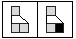it starts off from the string that represents True. And what one finds is that it generates exactly half of the possible strings of a given length. Let’s see, I have to figure out what the interpretation of this thing is—but anyway, it generates exactly half the strings of a given length, and you can apply negation to each of these strings and find out that it doesn’t generate the string that is the negation of that string.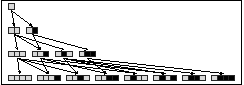OK. This  axiom system is both consistent and complete, but it’s fairly trivial. Well how about something that has all the richness of a real area of mathematics?

Well, Hilbert showed that Euclidean geometry is an example: something that is complete and consistent. And people thought in the early part of this century, that all other serious mathematical axiom systems would be the same way.

But what happened in 1931 was that Gödel showed that that wasn’t true. He showed that Peano arithmetic was incomplete—that there were propositions in Peano arithmetic that couldn’t be proved in any finite way.

Again, one might say, so what? I mean, in what’s called Robinson arithmetic one has a fine set of axioms, but it so happens that it’s fairly obvious that the system isn’t complete. Because, for example, you can’t prove that addition is commutative. So why is it a big deal that Peano arithmetic isn’t complete?

Well, it turns out that it’s really a quantitative issue, not a qualitative one.The point is that Peano arithmetic is very very nearly complete. I mean, all the simple propositions are connected—or their negations are connected—to the big network of all True propositions of arithmetic. But what Gödel showed is that there are some propositions that aren’t connected to this big network of true propositions of arithmetic. It’s a network that might look something like this, except that it grows much more rapidly at every step.

It’s a bit of a long story, but basically Gödel used the idea of universal computation to compile the statement "this statement is unprovable" into the very low-level machine code of Peano arithmetic, and that’s how  he got his result. But the machine code of that particular expression—the proposition in sort of algebraic terms that’s equivalent to "this statement is unprovable"— is incredibly long. So that’s what was so surprising about Gödel’s Theorem. That Peano arithmetic could seem almost complete—but not actually be complete.

Well, one might ask whether Gödel’s statement is the shortest statement that isn’t provable in Peano arithmetic. And it’s been known for some time that it’s not. There are some slightly simpler ones. But they’re still incredibly complicated. They’re nothing like Fermat’s Last Theorem, or some kind of question that mathematicians might seriously ask.

Well, as probably most of you know, Gödel’s Theorem was a big deal when it came out, because it showed that Hilbert’s ideas about mathematics, and about being able systematically to establish everything from axioms, weren’t going to work. But as a practical matter, in the past 68 or so years, nobody in practical mathematics has ended up paying all that much attention to Gödel’s Theorem. It’s always seemed too remote from the kind of questions that mathematicians actually end up asking. And the reason for this is that in Peano arithmetic the simplest incomplete propositions—that are known at least—are really complicated.

Well, I happen to think there are some somewhat simpler ones—I even have a potential candidate for one. But still, Peano arithmetic is basically a special almost-incompleteness-safe axiom system.

So… what does this mean about our efforts to find simple models of mathematics? Is this feature of Peano arithmetic something that’s very hard to get? And that requires all the hair of Peano arithmetic and that’s important in having something that is a realistic model of what mathematics could be.

One might think so. But it’s actually not true. Let me just say that if one searches through simple multiway systems, one can find other examples. Some systems are obviously inconsistent; some are obviously incomplete.

But it’s not hard to find ones where incompleteness happens sort of arbitrarily far away. So there’s nothing special about Peano arithmetic in this respect either.

So, OK. What are all these axiom systems that seem to have all the familiar math properties? Well, you’ve never heard of any of them. They’re just not things that have ever been reached in the history of human mathematics. And there’s a huge huge world of them out there. Some have easy interpretations to us humans. Some don’t. They’re sort of alternative mathematicses. So how does one compare these mathematicses to the mathematicses we’re familiar with?

I mean, each field of mathematics has a different character. Even within the mathematics that we are familiar with, different fields have different characters. How does that show up in the idealization of mathematics that I’ve been talking about?

One can think of building this network of theorems for each field. Say for number theory. Or geometry. Or functional analysis, or something like that. And then one could for example start asking simple questions like, what the statistical properties are. How connected it is? How many long paths there are in it?

Let me show you for instance for Euclid, Euclid’s Elements. This is the dependency graph of the propositions of Euclid’s Elements, in Book 1, Book 2, Book 3, etc.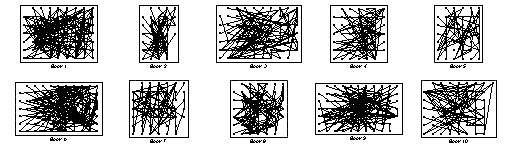You can unravel these to some extent. I haven’t got the best unravelling yet. Euclid was a little bit optimistic in what needed to have what depend on. But anyway, this is the network that you get from looking at the dependencies in Euclid’s Elements.

I was showing you this rather messy dependency network. This is the picture of the particular theorems Euclid thought were interesting that you get from the multiway system that is geometry. It reminds me of a quote from Gödel, when asked about mathematics, what the role of a mathematician was, his idea was that the role of the mathematician was to work out what theorems are interesting. That a machine can generate all the possible theorems, but the role of a mathematician is to work out what theorems should be considered interesting.

Anyway, what do the properties of this kind of network mean in mathematics? When people do mathematics they talk about deep theorems, powerful theorems, easy lemmas, elegant theorems those kinds of things. And it turns out that each of those kinds of things, one can start to try to define in a precise way in terms of the properties of a network like this.

And I think if one drew the networks for, say, number theory and say, algebra, one would be able to see by looking at them something about the very different characters of those fields.

So one question one might ask is, "What common features are there in the networks humans happen to have thought up for their mathematics?" I don’t exactly know right now. That’s something for which one would require quite a bit of empirical data to be able to work out. But the critical question is whether it’s all just historical, or whether some of it is a consequence of some general principle—or perhaps some human limitation or human foible.

It’s a little bit like asking about human languages, whether there are all possible forms that appear in human languages or not. The actual observation is that while there are incredibly many exceptions, typical human languages that people actually understand are not that far from being context free.

Well, it’s also interesting to think about what kind of mathematics . . . and thinking about whether the mathematics that we have is necessary, to think about what kind of mathematics the extraterrestrials might have. People tend to assume that they’ll think primes are important.

I think that’s a fairly absurd possibility. I’d bet on the rule 30 cellular automaton over the primes any day. And actually I think from my studies of all possible simple programs, I can say a certain amount about what’s likely to certainly exist in the mathematics of any creature. But it’s definitely not the primes, I think. I mean, even in human mathematical history, there are lots of obvious twists and turns. If you talked to Pythagoras, for instance, he’d be much more excited about perfect numbers than about prime numbers. But it so happens that primes have won out in the history of mathematics in the way it’s developed. But I don’t think that’s in the slightest bit fundamental.

But OK. If we think about building Mathematica, Mathematica does reflect actual history. I mean, we have all those special functions that happen to have been identified in the nineteenth century, and so on.

And they’re very useful. There’s nothing wrong with us pandering to the history of mathematics in designing Mathematica.

It’s just that it would be nice to find constructs that are as general as possible to represent what happens in humanly chosen mathematicses.

So that’s a challenge. Now, if one tries for example to do the proof thing in complete generality, and thinks that the idea of proof in general is the thing that is special about human mathematics, then one will quickly fail.

I mean, let’s say we try to make a function—call it say, FindRules—that takes a list of replacement rules, and instead of just having a single expression that it tries to reduce—it has two expressions, and tries to find a sequence of replacements from the list that it’s been given that will take one expression into the other.

Well, this function will be very unsatisfactory. It’ll have to work by doing the same kind of thing that we have to do in one of these multiway systems. And it will have a very hard time trying to find whether there happens to be a path from one of the expressions that we gave to the other expression that we gave.

We’d be getting tech support calls about this function all the time. And the reviewers would be doing benchmarks and complaining that the function is too slow, and so on. Because—and this is what I’ve discovered from looking at random simple programs— much of the time the network of things one can get to from the replacements is a big mess, and there’s no quick way to find out what’s going on.

In a sense, people would be reporting the undecidability of the halting problem as a bug all the time. But the question is: can one do better?

And there are two ways we might be able to do better. One is by using essentially a directly historical approach, and basing everything on the kinds of rules that happen to have shown up in arithmetic and geometry and all the things that have been derived from them.

But maybe, just maybe, one can do something more general. And to see if one can one has to understand what it really takes to be a human mathematics. I mean, if one looks at arbitrary mathematicses that come out from the simple rules and simple programs I’ve investigated, one is usually thrown into undecidability and so on very quickly. And in fact the whole idea of doing proofs and things pretty much disintegrates in that case.

But perhaps that isn’t true for any set of rules that would come from axioms that might realistically be used in human mathematics. It’s sort of the following question: if you see a random collection of axioms, can you tell if they had a human origin?

It’s again a little bit like asking about languages. If one has an approximate formal grammar for a language—a natural language or a computer language—can one tell if it was set up or used by humans? Or whether it was just randomly chosen?

I’m not sure about the answer to this for mathematics, and it’ll be interesting to see. One thing I am sure about is that if one doesn’t put on whatever constraints are associated with human mathematics, there’s an awful lot of other mathematicses out there to investigate. Mostly they can’t be investigated by the same methods as the ones that have been used so far in history in human mathematics. Mostly I think the proof idea for example won’t work. And instead one’s left with—just as in most fields of science—is mostly finding out what’s true by doing experiments, not by making proofs about what has to be true. And of course in doing those experiments that’s something that Mathematica can do very well in its present form.

But even though there’s a lot of other mathematicses out there that one might investigate—with all sorts of weird and wonderful properties—that doesn’t mean there’s no more to do with our regular old human mathematics.There certainly is. And I hope it can be done very well with Mathematica.

But as one tries to see how to do it, one has to understand what one is doing, and the fact that the mathematics that one is investigating is I think very much a historical, cultural, artefact. It happens to be a very wonderful artefact. Intellectually I think it’s much deeper than for example systems of human laws, or our schemes for art or architecture and things like that. But nevertheless an artefact.

And that means that one cannot somehow expect it to be typical of all the possible mathematicses, or of what might happen in nature.

But that’s what my new kind of science is trying to deal with: working out what all those possibilities are, what all possible mathematicses might do. And that’s what I’ve spent the past 8 years or so investigating, and in finding out how the mathematicses that are sampled by the natural world—what those are like and what their properties typically are.

Well, I should probably stop here. As is usual I’ve probably gone way over time. And I’m afraid this has gotten very abstract, but perhaps I’ve managed to communicate at least a little bit about a few of the things I’ve been thinking about. So I thank you all very much.